#?global_economy
#?aus_production
#?aus_livestock
#?hh_budget
tail(aus_retail)

5.1. Produce forecasts for the following series using whichever of NAIVE(y), SNAIVE(y) or RW(y ~ drift()) is more appropriate in each case:

Australian Population (global_economy)

aus.pop<-global_economy%>%filter(Country=="Australia")
aus.pop%>%autoplot(Population/1000000)+labs(x="Years", y="Population in Mlns", title= "Australian Population in millions")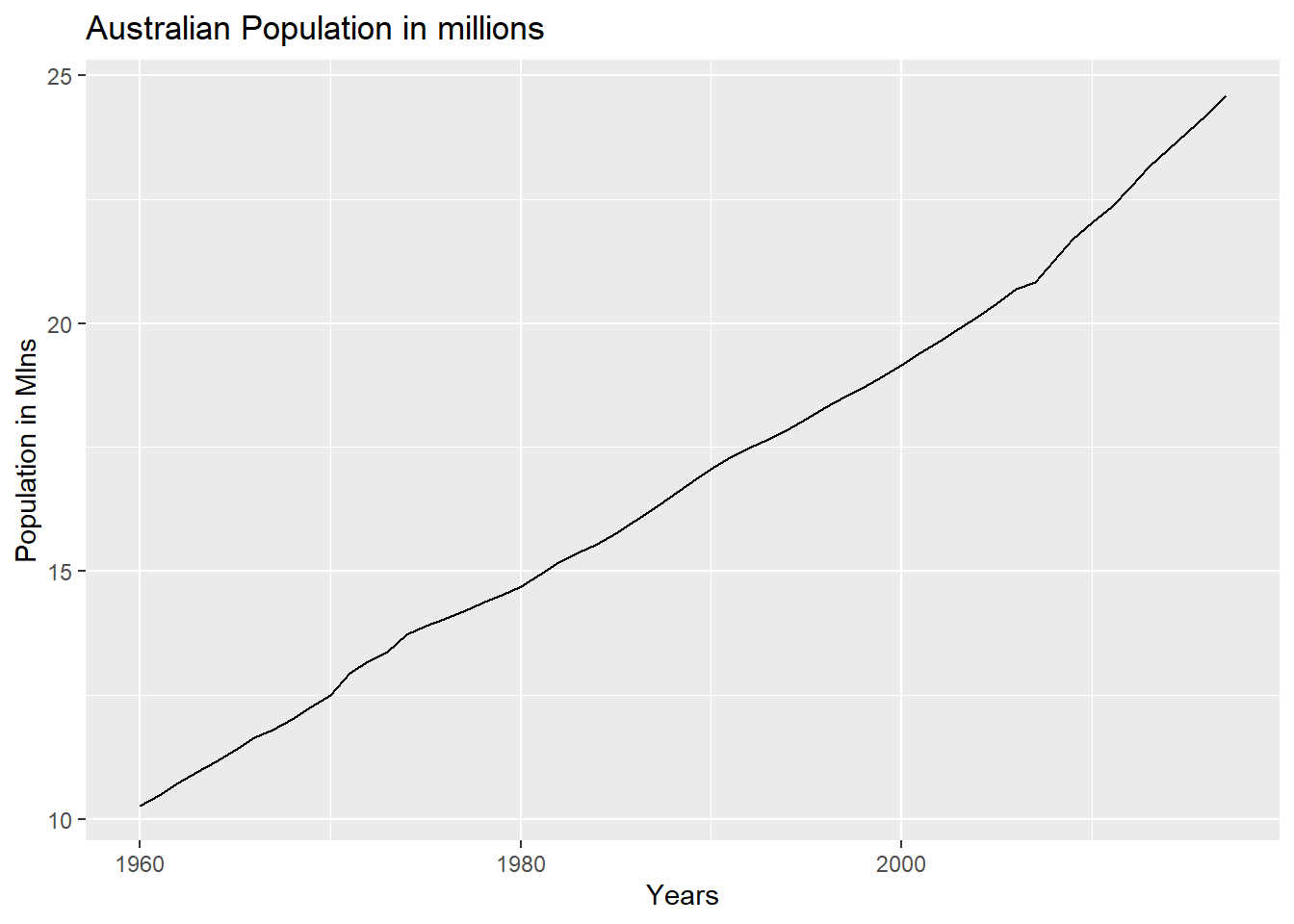From the above plot, we can see that the population has been increasing at a steady rate. Because of this, using the Naive and SNaive models would not be appropriate since the Naive model would just forecast based on the last known value and SNaive would incorporate seasonality which doesn’t really exist in the above. Using the Random walk model with a drift seems to be the best choice here.

aus.pop.fit<-aus.pop%>%model(RW(Population ~ drift()))
aus.pop.fc<-aus.pop.fit%>%forecast(h=5)

aus.pop.fc%>%autoplot(aus.pop)+ labs(title="Random Walk with drift - Forecast of Australian Population", subtitle = "h=5 years forecast", xlab="Years", ylab="Population")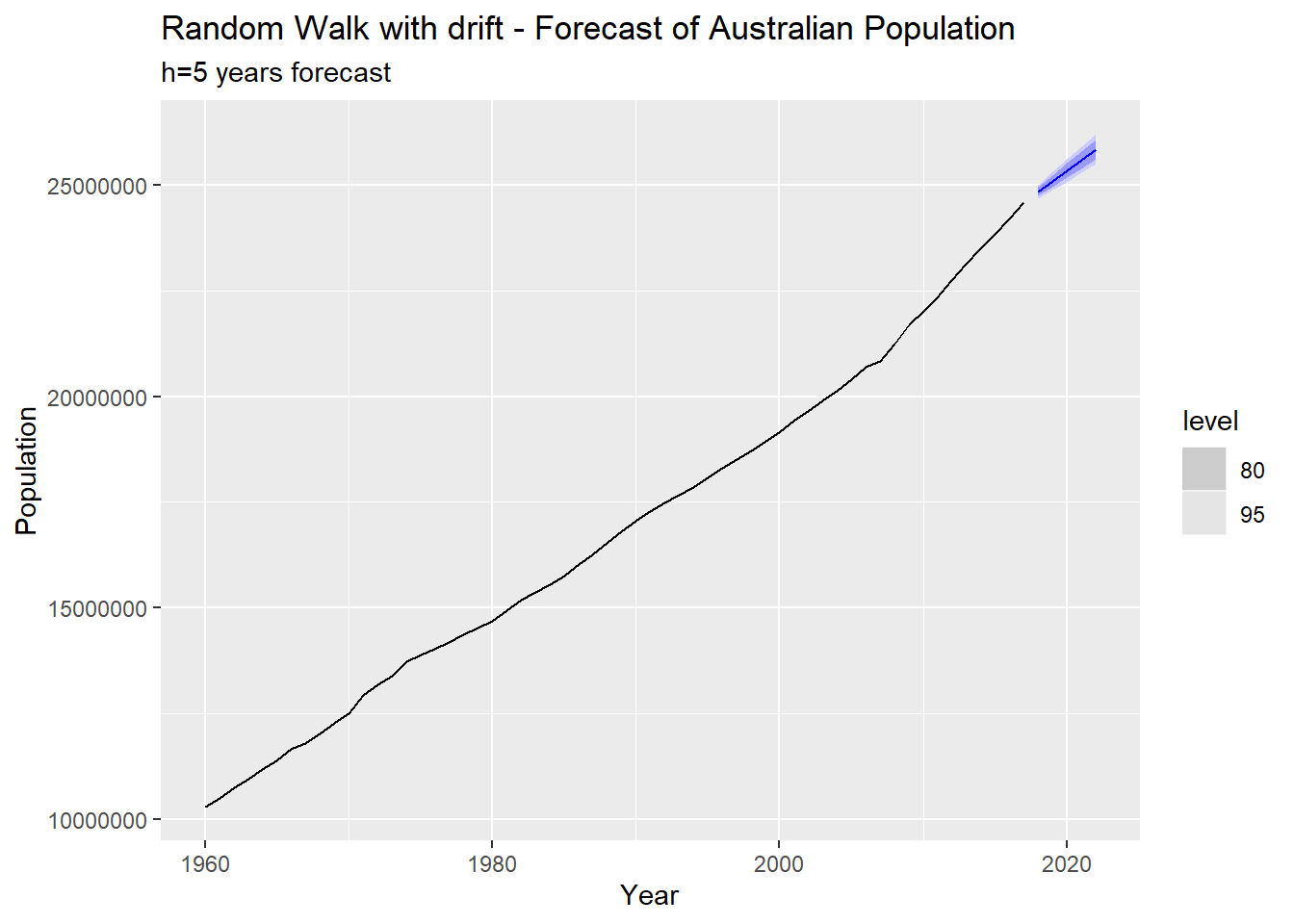Bricks (aus_production)

aus_production%>%autoplot(Bricks)
## Warning: Removed 20 row(s) containing missing values (geom_path).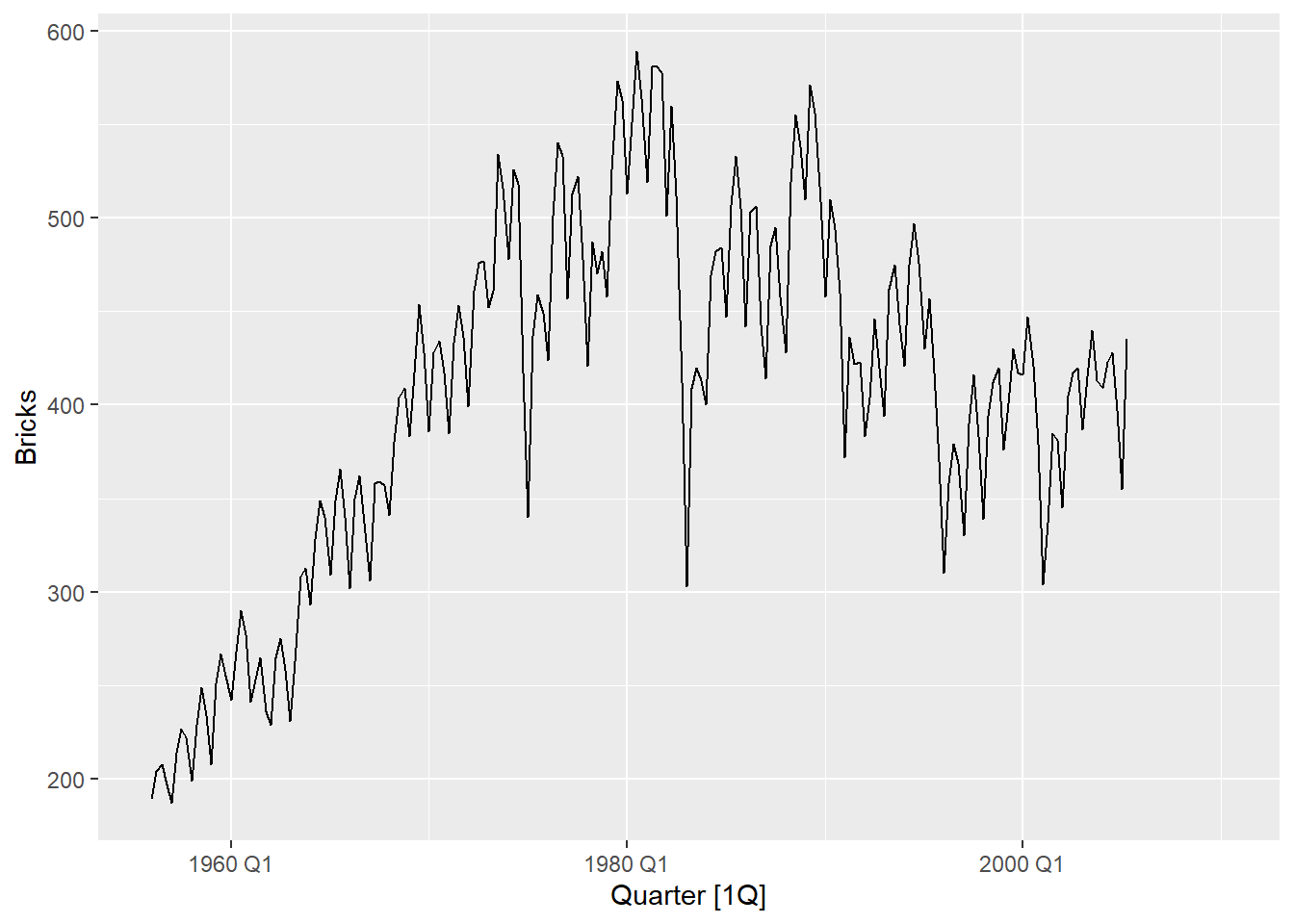From the plot above, it looks like there is seasonality in the production of bricks in Australia. We can generate the ggseason plot to verify this further. From the plot below, it looks like there is en element of seasonality wherein Q2 and Q3 production is typically higher than Q1 and Q4. Q1 is typically the lowest amount and Q3 is typically the highest amount.

So it would make sense to use the Seasonal Naive model with a lag = year, in this case.

aus_production%>%gg_season(Bricks)
## Warning: Removed 20 row(s) containing missing values (geom_path).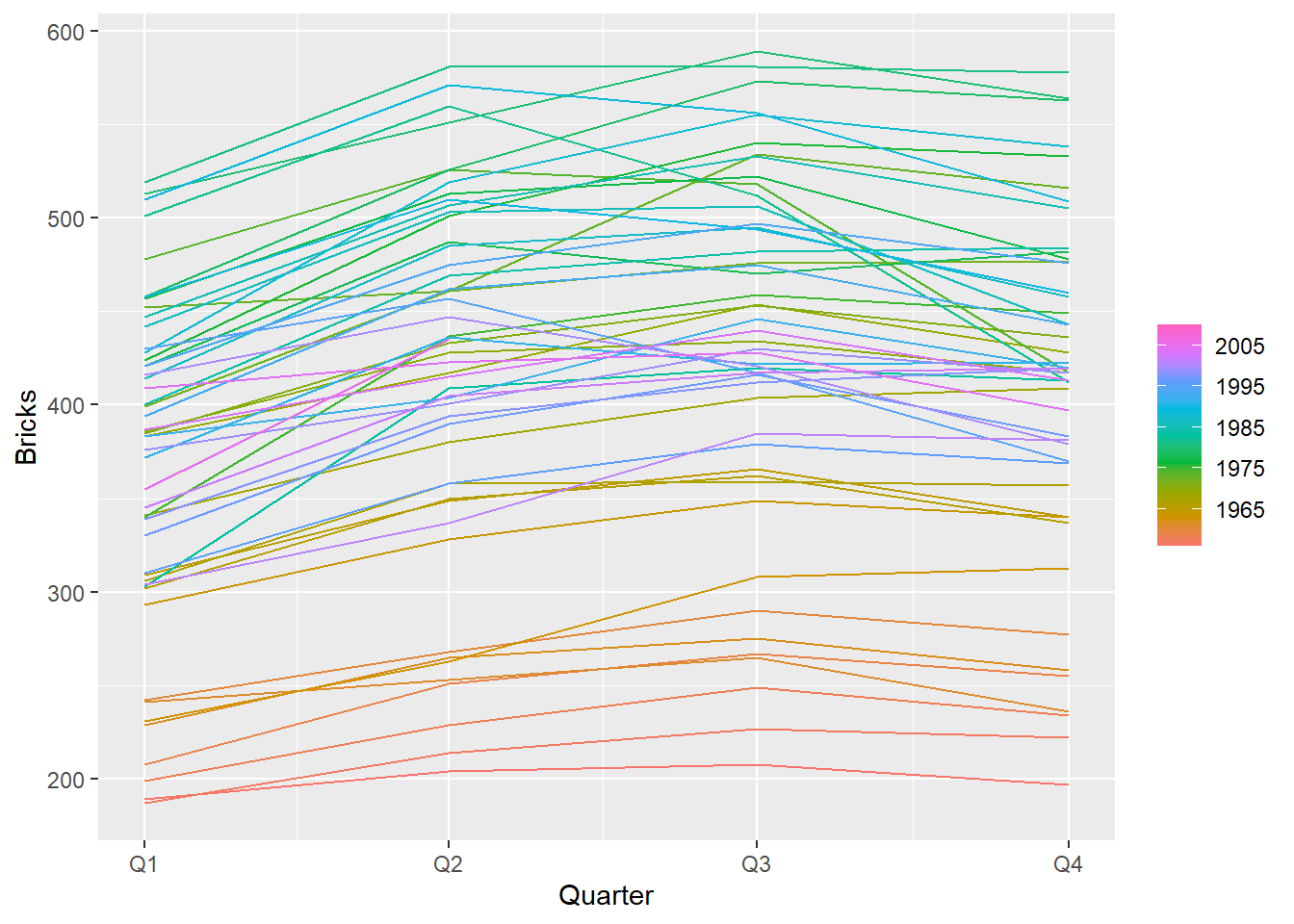bricks<-aus_production%>%drop_na()%>%select(Quarter, Bricks)

bricks.fit<-bricks%>%model(SNAIVE(Bricks~lag("year")))

bricks.fc<-bricks.fit%>%forecast(h=8)

bricks.fc%>%autoplot(bricks)+ labs(title="Forecast of Australian Brick production based on Seasonal Naive model", subtitle = "h=8 quarters forecast", xlab="Quarters", ylab="Bricks Production")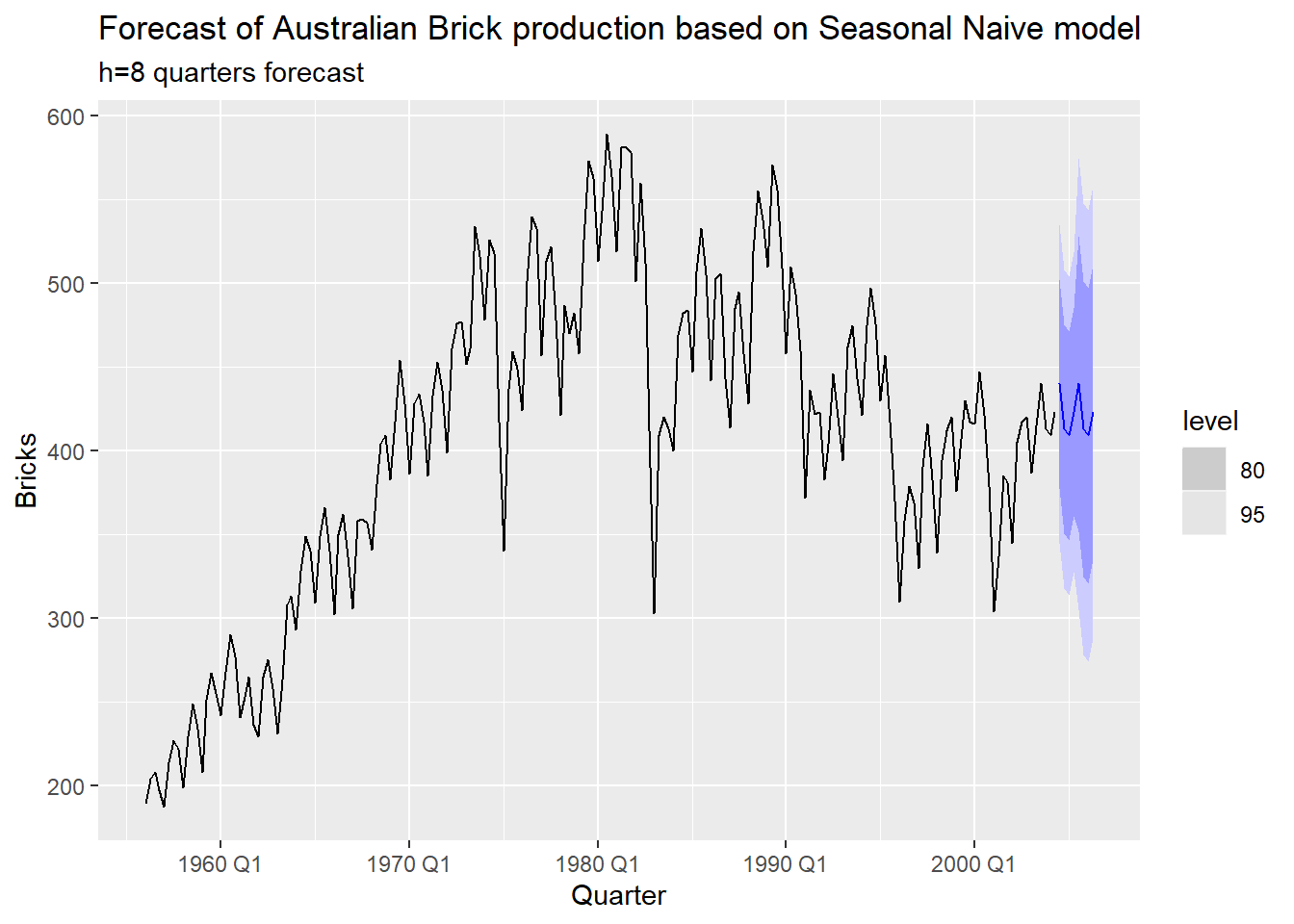NSW Lambs (aus_livestock)

nsw.lambs<-aus_livestock%>%filter(Animal=="Lambs",State=='New South Wales')%>%drop_na()
nsw.lambs%>%autoplot(Count)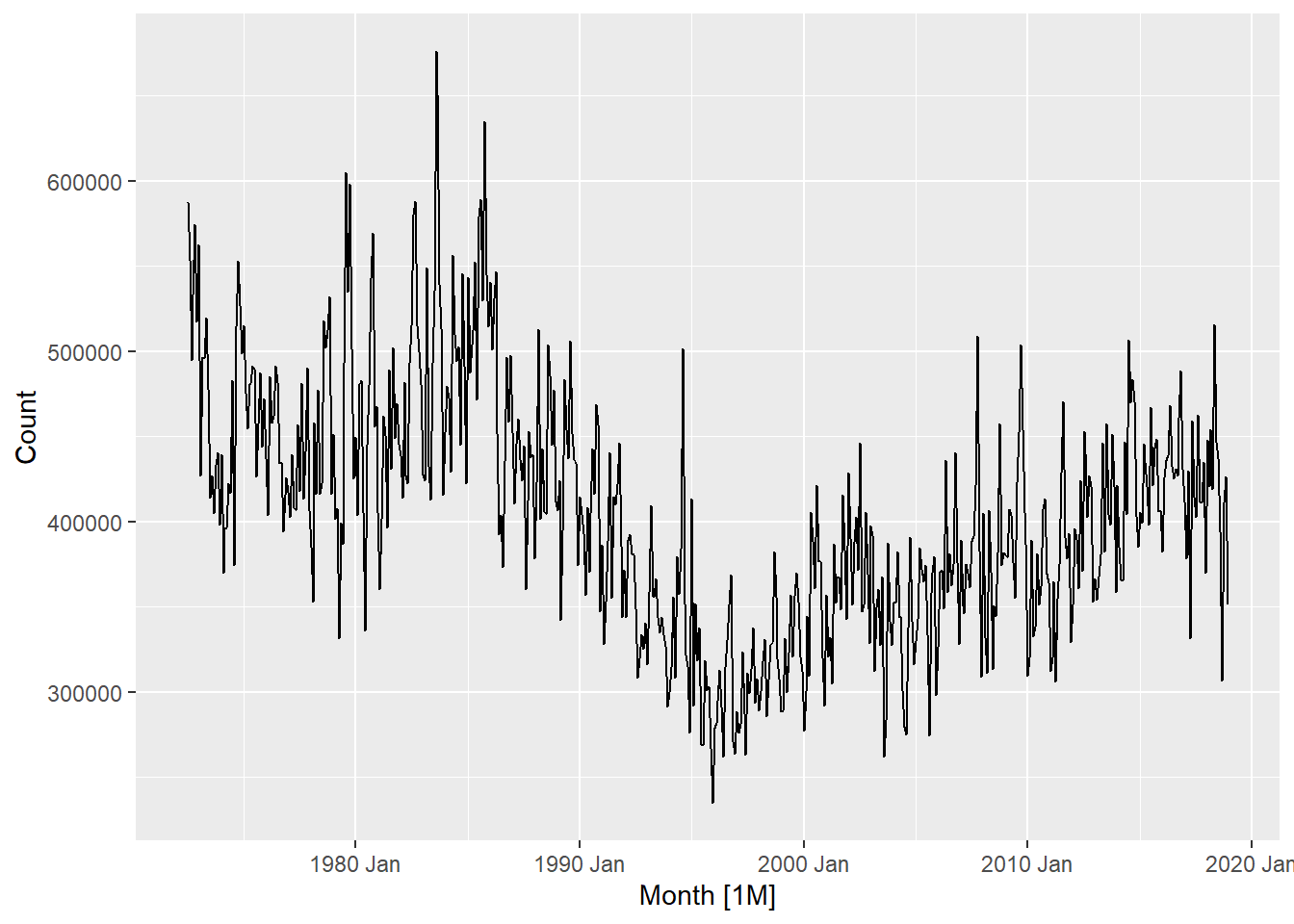From the plot above, we can see that the slaughter count seems to be seasonal in nature. To verify, we an plot the seasonal plot.

nsw.lambs%>%gg_season(Count)